Courses

# Bessel's Special Function (Part - 3) - Mathematical Methods of Physics, UGC - NET Physics Physics Notes | EduRev

## Physics for IIT JAM, UGC - NET, CSIR NET

Created by: Akhilesh Thakur

## Physics : Bessel's Special Function (Part - 3) - Mathematical Methods of Physics, UGC - NET Physics Physics Notes | EduRev

The document Bessel's Special Function (Part - 3) - Mathematical Methods of Physics, UGC - NET Physics Physics Notes | EduRev is a part of the Physics Course Physics for IIT JAM, UGC - NET, CSIR NET.
All you need of Physics at this link: Physics

HANKEL FUNCTIONS

Many authors prefer to introduce the Hankel functions by means of integral representations and then to use them to deﬁne the Neumann function Nν(z). An outline of this approach is given at the end of this section.

Deﬁnitions

Because we have already obtained the Neumann function by more elementary (and less powerful) techniques, we may use it to deﬁne the Hankel functions Hν(1) (x ) and Hν(2) (x ):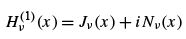(11.85)

and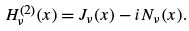(11.86)

This is exactly analogous to taking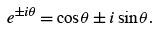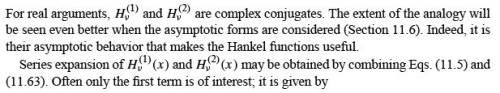Since the Hankel functions are linear combinations (with constant coefﬁcients) of Jν and Nν , they satisfy the same recurrence relations (Eqs. (11.10) and (11.12))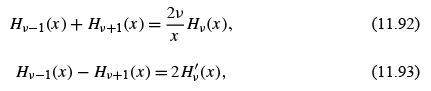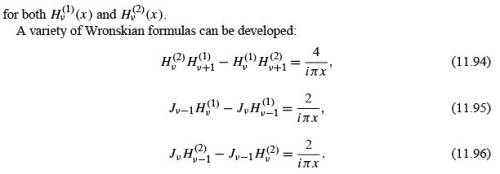Example 11.4.1 CYLINDRICAL TRAVELING WAVES

As an illustration of the use of Hankel functions, consider a two-dimensional wave problem similar to the vibrating circular membrane of Exercise 11.1.25. Now imagine that the waves are generated at r = 0 and move outward to inﬁnity. We replace our standing waves by traveling ones. The differential equation remains the same, but the boundary conditions change. We now demand that for large r the wave behave like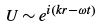(11.97)

to describe an outgoing wave. As before, k is the wave number. This assumes, for simplicity, that there is no azimuthal dependence, that is, no angular momentum, or m = 0. In Sections 7.3 and 11.6, H(1)(kr ) is shown to have the asymptotic behavior (for r →∞)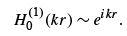(11.98)

This boundary condition at inﬁnity then determines our wave solution as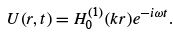(11.99)

This solution diverges as r → 0, which is the behavior to be expected with a source at the origin.

The choice of a two-dimensional wave problem to illustrate the Hankel function H0(1) (z) is not accidental. Bessel functions may appear in a variety of ways, such as in the separation of conical coordinates. However, they enter most commonly in the radial equations from the separation of variables in the Helmholtz equation in cylindrical and in spherical polar coordinates. We have taken a degenerate form of cylindrical coordinates for this illustration. Had we used spherical polar coordinates (spherical waves), we should have encountered index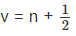,n an integer.

Contour Integral Representation of the Hankel Functions

The integral representation (Schlaeﬂi integral)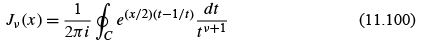may easily be established as a Cauchy integral for ν = n, an integer (by recognizing that the numerator is the generating function (Eq. (11.1)) and integrating around the origin).
If ν is not an integer, the integrand is not single-valued and a cut line is needed in our complex plane. Choosing the negative real axis as the cut line and using the contour shown in Fig. 11.7, we can extend Eq. (11.100) to nonintegral ν . Substituting Eq. (11.100) into Bessel’s ODE, we can represent the combined integrand by an exact differential that vanishes as t →∞e±iπ .
We now deform the contour so that it approaches the origin along the positive real axis, as shown in Fig. 11.8. For x> 0, this particular approach guarantees that the exact differential mentioned will vanish as t → 0 because of the e−x/2t → 0 factor. Hence each of the separate portions (∞ e−iπ to 0) and (0 to ∞ e ) is a solution of Bessel’s equation. We deﬁne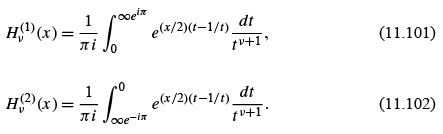These expressions are particularly convenient because they may be handled by the method of steepest descents. Hν(1) (x ) has a saddle point at t =+i , whereas Hν(2) (x ) has a saddle point at t =−i .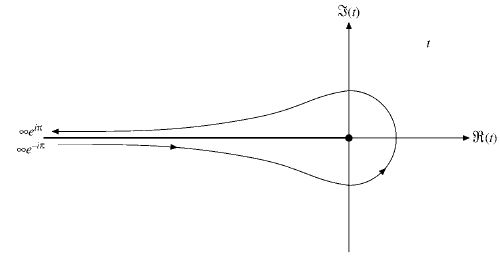FIGURE 11.7 Bessel function contour.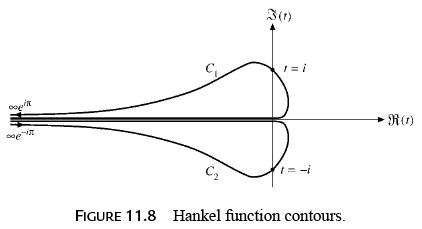The problem of relating Eqs. (11.101) and (11.102) to our earlier deﬁnition of the Hankel function (Eqs. (11.85) and (11.86)) remains. Since Eqs. (11.100) to (11.102) combined yield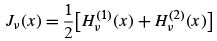(11.103)

by inspection, we need only show that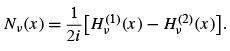(11.104)

This may be accomplished by the following steps :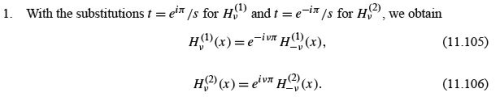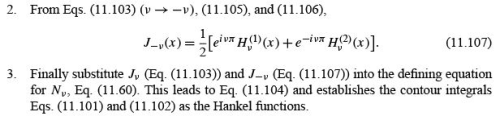Integral representations have appeared before: Eq. (8.35) for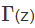and various representations of Jν (z). With these integral representations of the Hankel functions, it is perhaps appropriate to ask why we are interested in integral representations. There are at least four reasons. The ﬁrst is simply aesthetic appeal. Second, the integral representations help to distinguish between two linearly independent solutions. In Fig. 11.6, the contours C1 and C2 cross different saddle points. For the Legendre functions the contour for P(z) (Fig. 12.11) and that for Qn (z) encircle different singular points.

Third, the integral representations facilitate manipulations, analysis, and the development of relations among the various special functions. Fourth, and probably most important of all, the integral representations are extremely useful in developing asymptotic expansions. One approach, the method of steepest descents. A second approach, the direct expansion of an integral representation for the modiﬁed Bessel function Kν (z). This same technique may be used to obtain asymptotic expansions of the conﬂuent hypergeometric functions M and U .
In conclusion, the Hankel functions are introduced here for the following reasons:

• As analogs of e±ix they are useful for describing traveling waves.
• They offer an alternate (contour integral) and a rather elegant deﬁnition of Bessel functions.
• Hν(1) is used to deﬁne the modiﬁed Bessel function Kν.

MODIFIED BESSEL FUNCTIONS, Iν (x ) AND Kν (x )

The Helmholtz equation,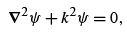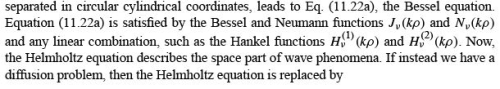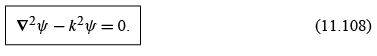The analog to Eq. (11.22a) is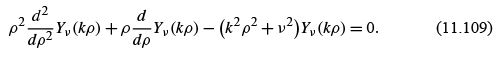The Helmholtz equation may be transformed into the diffusion equation by the transformation k → ik . Similarly, k → ik changes Eq. (11.22a) into Eq. (11.109) and shows that

Yν (kρ ) = Zν (i kρ ).

The solutions of Eq. (11.109) are Bessel functions of imaginary argument. To obtain a solution that is regular at the origin, we take Zν as the regular Bessel function Jν .Itis customary (and convenient) to choose the normalization so that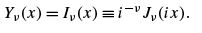(11.110)

(Here the variable kρ is being replaced by x for simplicity.) The extra i −ν normalization cancels the i ν from each term and leaves Iν (x ) real. Often this is written as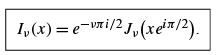(11.111)

I0 and I1 are shown in Fig. 11.10.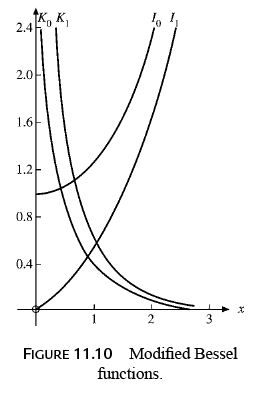Series Form

In terms of inﬁnite series this is equivalent to removing the (−1)s sign in Eq. (11.5) and writing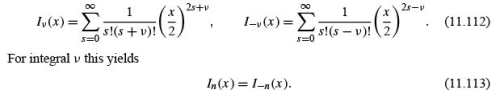Recurrence Relations

The recurrence relations satisﬁed by Iν (x ) may be developed from the series expansions, but it is perhaps easier to work from the existing recurrence relations for Jν (x ). Let us replace x by −ix and rewrite Eq. (11.110) as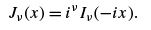(11.114)

Then Eq. (11.10) becomes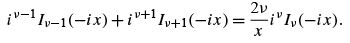Replacing x by ix , we have a recurrence relation for Iν (x ),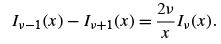(11.115)

Equation (11.12) transforms to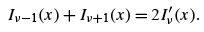(11.116)

These are the recurrence relations. It is worth emphasizing that although two recurrence relations, Eqs. (11.115) and (11.116) or  specify the second-order ODE, the converse is not true. The ODE does not uniquely ﬁx the recurrence relations. Equations (11.115) and (11.116)  provide an example.
From Eq. (11.113) it is seen that we have but one independent solution when ν is an integer, exactly as in the Bessel functions Jν . The choice of a second, independent solution of Eq. (11.108) is essentially a matter of convenience. The second solution given here is selected on the basis of its asymptotic behavior — as shown in the next section. The confusion of choice and notation for this solution is perhaps greater than anywhere else in this ﬁeld.  Many authors choose to deﬁne a second solution in terms of the Hankel function Hν(1) (x ) by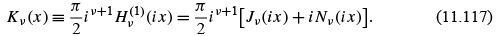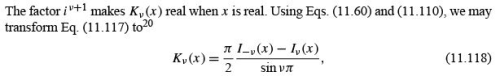analogous to Eq. (11.60) for Nν (x ). The choice of Eq. (11.117) as a deﬁnition is somewhat unfortunate in that the function Kν (x ) does not satisfy the same recurrence relations as Iν (x ) . zTo avoid this annoyance, other authors21 have included an additional factor of cos νπ . This permits Kν to satisfy the same recurrence relations as Iν , but it has the disadvantage of making Kν = 0for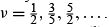The series expansion of Kν (x ) follows directly from the series form of Hν(1) (i x ).The lowest-order terms are (cf. Eqs. (11.61) and (11.62))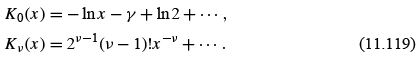Because the modiﬁed Bessel function Iν is related to the Bessel function Jν , much as sinh is related to sine, Iν and the second solution Kν are sometimes referred to as hyperbolic Bessel functions. K0 and K1 are shown in Fig. 11.10.
I0 (x ) and K0 (x ) have the integral representations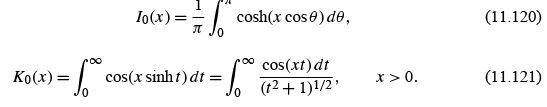Equation (11.120) may be derived from Eq. (11.30) for J0 (x ) or may be taken as  ν = 0. The integral representation of K0 , Eq. (11.121), is a Fourier transform and may best be derived with Fourier transforms, Chapter 15, or with Green’s functions. A variety of other forms of integral representations (including ν ≠ 0) appear in the exercises. These integral representations are useful in developing asymptotic forms  and in connection with Fourier transforms.
To put the modiﬁed Bessel functions Iν (x ) and Kν (x ) in proper perspective, we introduce them here because:

•  These functions are solutions of the frequently encountered modiﬁed Bessel equation.
• They are needed for speciﬁc physical problems, such as diffusion problems.
• Kν (x ) provides a Green’s function.
• Kν (x ) leads to a convenient determination of asymptotic behavior .
Offer running on EduRev: Apply code STAYHOME200 to get INR 200 off on our premium plan EduRev Infinity!

159 docs

,

,

,

,

,

,

,

,

,

,

,

,

,

,

,

,

,

,

,

,

,

,

,

,

;